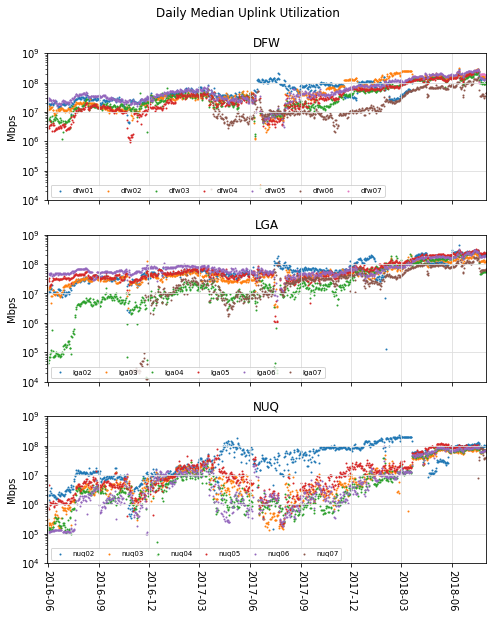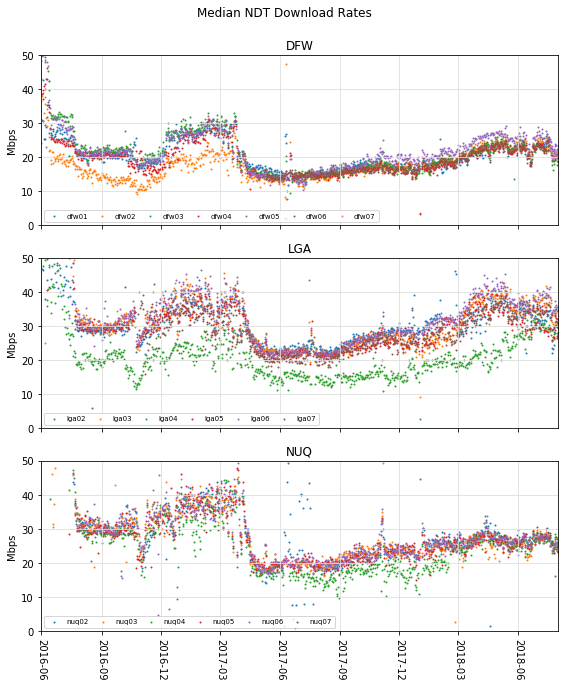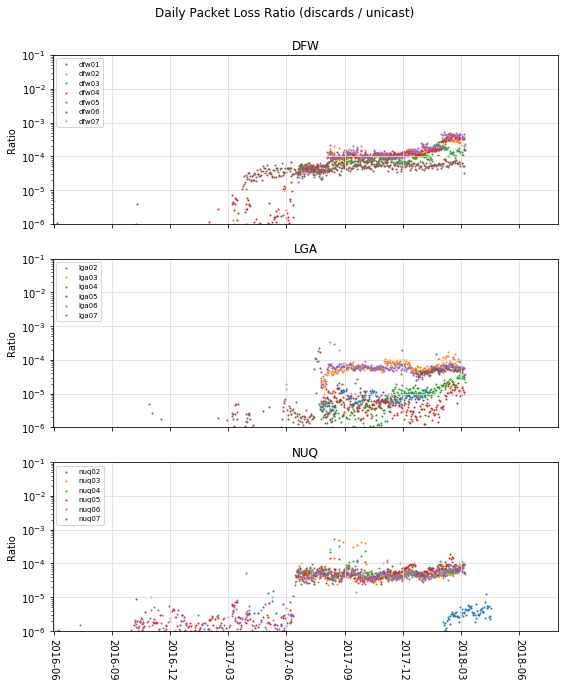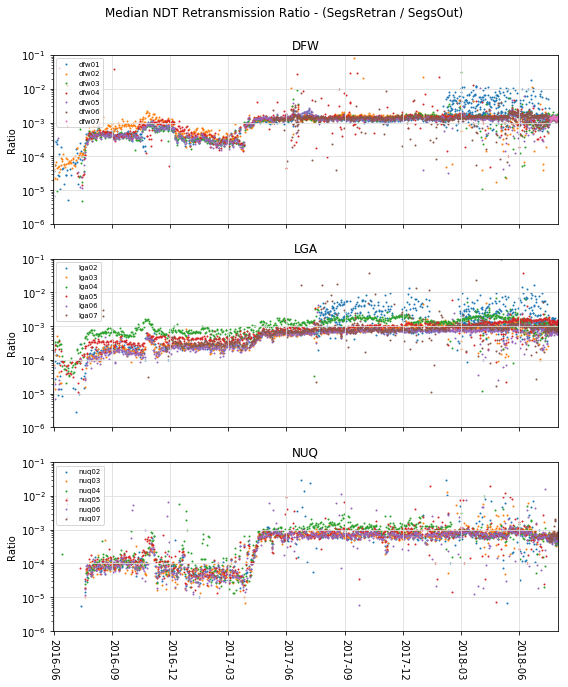MeasurementLab

# Abstract¶

On February 1st, 2018, during a regular data quality review, we identified an increase in switch discards at sites with 10Gbps equipment connected to 1Gbps uplinks. We have collected high-resolution, switch telemetry (DISCO) since June 2016 to monitor traffic microbursts. To assess the impact of switch discards at 10Gbps sites, we deployed a new ETL parser for the DISCO data set. By February 15th, we found that affected sites had up to 30% of timebins with discard counts greater than zero. Immediately, we began trials to test remediation strategies. After identifying Ethernet flow control as an option, we began live trials the week of the 26th. And, by March 8th, all affected sites were enabled with Ethernet flow control. The rate of switch discards at sites with 10Gbps equipment is now zero. Since April 13th, the DISCO dataset includes pause frame counts to observe Ethernet flow-control activity.

The rate of switch discards was approximately 10x less than the rate of retransmissions found in NDT download tests. In other words, the Internet has a 10x higher discard rate than the M-Lab switch in the worst case. When we compare download performance before and after enabling Ethernet flow-control, we find no measurable performance impact of switch discards on the NDT data set.

This report:

• describes the cause of switch discards.
• describes the analysis used to conclude that the configuration change was positive.
• describes how to determine whether experiment data was affected by switch discards.

# Timeline¶

2018-02-01:

• During data-quality review, we identify high levels of discards in SNMP monitoring.
• We prioritize parsing high-resolution switch data for detailed investigation.

2018-02-15:

• The DISCO data confirms excessive packet discard rates at many sites.
• We recognize that this is due to 10g hardware with 1g uplinks.
• We begin investigating options for remediation.

2018-02-22 - 27:

• Test & Live trials exploring software solutions: DPDK and qdisc

2018-03-01:

• We recognize that Ethernet flow-control is a better solution than qdisc.
• Live trials using Ethernet flow-control begin.

2018-03-06 - 08:

• We enable flow-control at all 10g sites.

2018-04-13:

• DISCO data set includes pause-frame counts globally.

# Remediation: Enable Ethernet Flow-Control¶

By design, network devices may discard packets as a signal to senders that the network is under congestion. In fact, some technologies seeking to reduce packet discards turn out to negatively affect individual or aggregate network performance, i.e. head-of-line blocking, buffer-bloat. As a measurement platform, M-Lab seeks to balance measurement fidelity of actual network conditions with test performance under those conditions.

To prevent packet discards in M-Lab switches, we have enabled Ethernet flow-control on all 10Gbps machine-to-switch ports. Ethernet flow-control prevents switch discards due to tail drop in the single-machine, 10Gbps-to-1Gbps configuration. As well, Ethernet flow-control prevents switch discards due to fan-in congestion in the multi-machine, 10Gbps-to-10Gbps configuration. Both scenarios introduce no more contention between machines than already exists from the uplink capacity.

While Ethernet flow-control is known to cause “head of line blocking” in certain circumstances, these do not apply to M-Lab sites. In particular, M-Lab sites are optimized for sending “north-south” traffic (e.g. upload / download through the switch) and never “east-west” traffic (e.g. between machines). This eliminates all sources of “external head of line blocking” from machine-to-machine traffic. As well, our configuration enables flow-control only between the machine-to-switch link, so cannot contribute to “congestion spreading” beyond the switch. Because Ethernet flow-control can contribute to problems in more complex configurations, "everybody knows" that it should not be turned on. However, M-Lab's unique setup makes Ethernet flow-control helpful.

Since 2016-06, the DISCO data set has recorded switch telemetry at 10 second intervals, including packet discards. Since enabling Ethernet flow-control the occurrence of packet discards has reduced to zero. In 2018-04, we added pause-frame counters to the DISCO data set. The pause-frame counters allow us to observe flow-control in action, and we have not observed bad NDT performance correlated with Ethernet flow-control.

# Analysis¶

As M-Lab client integrations have grown over time, so has the percentage of site uplink capacity. Figure 1 shows the increase of median uplink utilization over a two year period using unicast transfer rates. Between late 2016 and late 2018, the daily median transfer rates have increased roughly 10x, more in some sites. Figure 2 shows the corresponding change in the median NDT download rates over the same period.

In :
# To load figures outside browser, use "%matplotlib" with no argument.
%matplotlib inline

import os
import math
import pandas as pd
import numpy as np
import matplotlib
import matplotlib.dates as dates
import matplotlib.pyplot as plt
import matplotlib.ticker
import datetime
import collections
import itertools

from scipy import stats

# Depends on: pip install sklearn
from sklearn.model_selection import train_test_split

# Some matplotlib features are version dependent.
assert(matplotlib.__version__ >= '2.1.2')

def run_query(query, project='measurement-lab'):
client = bigquery.Client(project=project)
job = client.query(query)

results = collections.defaultdict(list)
for row in job.result(timeout=3000):
for key in row.keys():
results[key].append(row.get(key))

return pd.DataFrame(results)

def unlog(x, pos):
"""Formats the x axis for histograms taken on the log of values."""
v = math.pow(10, x)
frac, whole = math.modf(v)
if frac > 0:
return '%.1f' % v
else:
return '%d' % whole

def hist(vals, bin_count, log=True, cdf=False):
"""Produces hist or cdf values for smooth plots."""
if log:
r = [math.log10(x) for x in vals]
else:
r = vals

m, bins = np.histogram(r, bin_count, normed=True)
m = m.astype(float)

tops = m
if cdf:
tops = np.cumsum(m)
total = sum(m)
tops = [float(t) / total for t in tops]

matplotlib.rcParams['figure.dpi']= 150
logFormatter = matplotlib.ticker.FuncFormatter(unlog)

In :
def plot_df(
df, xname='', yname='',
cname='', bins=None, cdf=False,
xlog=None, ylog=False,
fig_by='', axes_by='', group_by='',
figsize=(6,8), axes=(1,1),
label='{group}',
xlabel='', ylabel='',
xlim=(), ylim=(),
fx=list, fy=list,
suptitle='',
title='',
legend={},
figmap=None,
fxn=None,
info=False):
"""Creates a scatter or histogram plot from df, split on mulitple dimension.

plot_df helps plot structured data frames as simple scatter or histograms by
slicing the dataframe along distinct values of some column names. For
example, a df that includes a "state" column could be used to create a new
figure for every state with the fig_by="state" parameter. Within a single
figure, it's possible to slice the data into multiple axes using another
column, for example one named "city" using axes_by="city".

Args:
df: pandas.DataFrame, structured data to plot.
xname: str, name of df column to use as x-axis. Use only with yname.
yname: str, name of df column to use as y-axis. Use only with xname.
cname: str, name of df column to calculate the histogram. Use only with
cdf, and bins.
cdf: bool, whether to plot histogram as a CDF. Default is as a PDF. Use
only with cname and bins.
bins: int or callable, the number of histogram bins. May be a function.
xlog: bool, whether to take the log of histogram. Use only with cname.
ylog: bool, whether to plot the y axis using semilog scale.
fig_by: str, name of column where distinct values split data into multiple
figures.
axes_by: str, name of column where distinct values split data into
multiple axis panels on a single figure.
group_by: str, name of column where distinct values are all plotted on the
same axis.
figsize: (int, int), dimensions of figure. Default (6, 8).
axes: (int, int), arrangement of axes within figure. Default (1, 1).
label: str, the legend format per data series. Used as a format string.
Other parameters available are {figure}, {axis}, {size}.
Default {group}.
xlabel: str, the xlabel value. Used as a format string. Other parameters
available are {figure}, {axis}, {size}.
ylabel: str, the ylabel value. Used as a format string like xlabel.
xlim: (xmin, xmax), explicitly set minimum and maximum values of x axis.
ylim: (ymin, ymax), explicitly set minimum and maximum values of y axis.
fx: func, if set, operate on x axis series data before plotting.
fy: func, if set, operate on y axis series data before plotting.
suptitle: str, figure title.
title: str, axis title.
legend: **legend_args,
figmap: the figmap value returned by an earlier cal of plot_df. May be
used to overlay values from multiple data frames. Must use the same
fig_by, axes_by, and group_by values.
fxn: callable that accepts parameters (r, **kwargs). Kwargs will include
figure, axis, group, names and data set size. Only called for
histogram plots.
info: bool, whether to log additional info messages.
Returns:
dict of str to (figures, axes) tuples
"""
def log_info(f):
if info:
print f

def get_label_color(ax, label):
"""Returns the color of the collection with given label."""
color = None
for c in ax.collections:
if c.get_label() == label:
color = c.get_facecolors()
return color

check_colors = figmap is not None
if figmap is None:
log_info('new figmap')
figmap = {}

scatter = None
if (xname and yname):
scatter = True
if cname:
scatter = False
if scatter is None:
raise Exception('Provide xname and yname or cname')

default_names = set(['default'])

figure_names = set(df[fig_by]) if fig_by else default_names
for f in sorted(figure_names):
if f in figmap:
fig, ax, ax_index = figmap[f]
else:
fig = plt.figure(figsize=figsize)
ax = fig.subplots(axes, axes, squeeze=False)
ax_index = list(itertools.product(range(axes), range(axes)))
log_info('saving figmap for %s' % f)
figmap[f] = (fig, ax, ax_index)

df_fig = df if f == 'default' else df[df[fig_by] == f]

axes_names = set(df_fig[axes_by]) if axes_by else default_names
for p, a in enumerate(sorted(axes_names)):
if p >= len(ax_index):
print 'SKIPPING', p, f, a, 'too few axes positions'
continue

if a == 'default':
df_axes = df_fig
else:
df_axes = df_fig[df_fig[axes_by] == a]

i, j = ax_index[p]
group_names = set(df_axes[group_by]) if group_by else default_names
for g in sorted(group_names):
if g == 'default':
df_g = df_axes
else:
df_g = df_axes[df_axes[group_by] == g]

if scatter:
x = fx(df_g[xname])
y = fy(df_g[yname])
l = label.format(figure=f, axis=a, group=g)
kw = {}
found_color = None
if check_colors:
# When a figmap is given, and the current label matches
# an existing label, re-use the original color.
found_color = get_label_color(ax[i][j], l)

if found_color is not None:
kw['color'] = found_color
else:
kw['label'] = l

ax[i][j].scatter(x, y, s=1, **kw)

else:
r = df_g[cname]
if bins is None:
size = int(math.sqrt(len(r)))
else:
size = bins(r)
if fxn:
result = fxn(r, figure=f, axis=a, group=g, size=size)
log_info("%s %s %s %s %s" % (f, a, g, size, len(r)))
h_tops, h_bins = hist(r, size, log=xlog , cdf=cdf)
l = label.format(figure=f, axis=a, group=g, size=size,
result=result)
ax[i][j].plot(h_bins[:-1], h_tops, label=l)

if i != len(ax)-1:
ax[i][j].set_xticklabels([])

if title:
ax[i][j].set_title(title.format(figure=f, axis=a, group=g))
if ylabel:
ax[i][j].set_ylabel(ylabel.format(figure=f, axis=a, group=g))
if xlabel:
ax[i][j].set_xlabel(xlabel.format(figure=f, axis=a, group=g))

if xlim:
ax[i][j].set_xlim(xlim)
if ylim:
ax[i][j].set_ylim(ylim)

ax[i][j].grid(color='#dddddd')
ax[i][j].legend(fontsize='x-small', **legend)
if scatter:
ax[i][j].tick_params(axis='x', labelrotation=-90)
if xlog:
ax[i][j].xaxis.set_major_formatter(logFormatter)
if ylog:
ax[i][j].semilogy()

if suptitle:
fig.suptitle(suptitle.format(figure=f))
fig.tight_layout(rect=[0, 0.03, 1, 0.95])

return figmap

def plot_scatter(df, xname, yname, **kwargs):
return plot_df(df, xname=xname, yname=yname, **kwargs)

def plot_hist(df, cname, bins=None, **kwargs):
return plot_df(df, cname=cname, bins=bins, **kwargs)


In :
df_disco_pct = run_query("""
#standardSQL

WITH measurementlab_switch_dedup AS (
SELECT
metric,
REGEXP_EXTRACT(hostname, r'(mlab[1-4].[a-z]{3}[0-9]{2}).*') AS hostname,
sample.timestamp AS ts,
sample.value AS value
FROM
measurement-lab.base_tables.switch*,
UNNEST(sample) AS sample
WHERE
AND REGEXP_CONTAINS(hostname, r"mlab1.(dfw|lga|nuq)\d\d")
GROUP BY
hostname, metric, ts, value
)

SELECT
UPPER(REGEXP_EXTRACT(hostname, r'mlab1.([a-z]{3})[0-9]{2}.*')) AS metro,
REGEXP_EXTRACT(hostname, r'mlab1.([a-z]{3}[0-9]{2}).*') AS site,
UNIX_SECONDS(TIMESTAMP_TRUNC(ts, DAY)) AS ts,
0.8 * APPROX_QUANTILES(value, 101)[ORDINAL(50)] as bytes_50th

FROM
measurementlab_switch_dedup

WHERE
hostname IS NOT NULL

GROUP BY
hostname, ts

ORDER BY
hostname, ts
""")

In :
_ = plot_scatter(
df_disco_pct, 'ts', 'bytes_50th',
axes_by='metro', group_by='site',
axes=(3, 1), figsize=(7, 9),
ylabel="Mbps",
title='{axis}',
xlim=(pd.to_datetime("2016-05-31"), pd.to_datetime("2018-08-01")),
ylim=(1e4, 1e9),
fx=lambda l: [pd.to_datetime(t, unit='s') for t in l],
legend={'loc':3, 'ncol':7, 'columnspacing':1},
ylog=True)Figure 1: Daily Median Uplink Utilization. Rates from select US sites from 2016-06 to 2018-08. Rates include all unicast packets (UDP & TCP) according to the DISCO data set.

In :
df_ndt_all = run_query("""
WITH measurementlab_ndt_dedup AS (
SELECT
connection_spec.server_hostname as server_hostname,
web100_log_entry.connection_spec.remote_ip as remote_ip,
log_time,
(8 * (web100_log_entry.snap.HCThruOctetsAcked / (
web100_log_entry.snap.SndLimTimeRwin +
web100_log_entry.snap.SndLimTimeCwnd +

FROM
measurement-lab.release.ndt_all

WHERE
REGEXP_CONTAINS(connection_spec.server_hostname, r"(lga|dfw|nuq)\d\d")
AND web100_log_entry.snap.HCThruOctetsAcked >= 1000000
AND (web100_log_entry.snap.SndLimTimeRwin +
web100_log_entry.snap.SndLimTimeCwnd +
web100_log_entry.snap.SndLimTimeSnd) >= 9000000
AND (web100_log_entry.snap.SndLimTimeRwin +
web100_log_entry.snap.SndLimTimeCwnd +
web100_log_entry.snap.SndLimTimeSnd) < 600000000
AND connection_spec.data_direction = 1
AND web100_log_entry.connection_spec.remote_ip != "45.56.98.222"
AND web100_log_entry.connection_spec.remote_ip != "2600:3c03::f03c:91ff:fe33:819"
AND web100_log_entry.connection_spec.remote_ip != "35.225.75.192"
AND web100_log_entry.connection_spec.remote_ip != "35.192.37.249"
AND web100_log_entry.connection_spec.remote_ip != "35.193.254.117"
AND log_time >= TIMESTAMP("2016-06-01")

GROUP BY
connection_spec.server_hostname,
log_time,
web100_log_entry.connection_spec.remote_ip,
web100_log_entry.connection_spec.local_ip,
web100_log_entry.connection_spec.remote_port,
web100_log_entry.connection_spec.local_port,
)

SELECT
metro,
site,
day,
count(*) as count

FROM
(
SELECT
UPPER(REGEXP_EXTRACT(server_hostname, r"([a-z]{3})[0-9]{2}")) as metro,
REGEXP_EXTRACT(server_hostname, r"([a-z]{3}[0-9]{2})") as site,
TIMESTAMP_TRUNC(log_time, DAY) as day,

FROM
measurementlab_ndt_dedup

GROUP BY
metro, site, day, remote_ip
)

GROUP BY
metro, site, day

ORDER BY
day
""")

In :
_ = plot_scatter(
axes_by='metro', group_by='site',
axes=(3, 1), figsize=(8, 10),
ylabel="Mbps",
title='{axis}',
xlim=(pd.to_datetime("2016-05-31"), pd.to_datetime("2018-08-01")),
ylim=(0, 50),
fx=lambda l: [pd.to_datetime(t) for t in l],
legend={'loc':3, 'ncol':7, 'columnspacing':1})Figure 2: Daily median NDT download rates from select US sites from 2017-05 to 2018-08. Multiple tests from the same remote_ip are counted only once. Figure 2 shows median client behavior changing over time. These client behavior changes started before the hardware upgrades (so are unaffected by the switch discards).

# Daily Packet Loss Ratio¶

In :
df_disco_ratio = run_query("""
#standardSQL

WITH measurementlab_switch_dedup AS (
SELECT
metric,
REGEXP_EXTRACT(hostname, r'(mlab[1-4].[a-z]{3}[0-9]{2}).*') AS hostname,
sample.timestamp AS ts,
sample.value AS value

FROM
measurement-lab.base_tables.switch*,
UNNEST(sample) AS sample

WHERE
AND REGEXP_CONTAINS(hostname, r"mlab1.(dfw|lga|nuq)\d\d")

GROUP BY
hostname, metric, ts, value
)

SELECT
UPPER(REGEXP_EXTRACT(hostname, r'mlab1.([a-z]{3})[0-9]{2}.*')) AS metro,
REGEXP_EXTRACT(hostname, r'mlab1.([a-z]{3}[0-9]{2}).*') AS site,
hostname,
ts,
IF(total > 0, discards / total, 0) as ratio

FROM (
SELECT
hostname,
UNIX_SECONDS(TIMESTAMP_TRUNC(ts, DAY)) AS ts,
SUM(IF(metric = "switch.unicast.uplink.tx", value, 0)) AS total
FROM
measurementlab_switch_dedup
WHERE
hostname IS NOT NULL
GROUP BY
hostname, ts
HAVING
ORDER BY
hostname, ts
)
GROUP BY
hostname, ts, ratio
HAVING
ratio < 0.01
ORDER BY
hostname, ts
""")

In :
_ = plot_scatter(
df_disco_ratio, 'ts', 'ratio',
axes_by='metro', group_by='site',
axes=(3, 1), figsize=(8, 10),
suptitle='Daily Packet Loss Ratio (discards / unicast)',
ylabel="Ratio",
title='{axis}',
xlim=(pd.to_datetime("2016-05-31"), pd.to_datetime("2018-08-01")),
ylim=(1e-6, 1e-1),
fx=lambda l: [pd.to_datetime(t, unit='s') for t in l],
legend={'loc':2},
ylog=True)Figure 3: Switch Discard Ratio calculated using the daily switch uplink discard count divided by the total uplink packet count. DFW had the globally highest rate of discards. Typically other sites were less than 0.01% (1 packet per 10,000 packets).

# Median NDT Retransmission Ratio¶

In :
df_ndt_retrans = run_query("""
WITH measurementlab_ndt_dedup AS (
SELECT
connection_spec.server_hostname as hostname,
web100_log_entry.connection_spec.remote_ip as remote_ip,
log_time,
web100_log_entry.snap.SegsRetrans as SegsRetrans,
web100_log_entry.snap.SegsOut as SegsOut

FROM
measurement-lab.release.ndt_all

WHERE
REGEXP_CONTAINS(connection_spec.server_hostname, r"(lga|dfw|nuq)\d\d")
AND web100_log_entry.snap.HCThruOctetsAcked >= 1000000
AND (web100_log_entry.snap.SndLimTimeRwin +
web100_log_entry.snap.SndLimTimeCwnd +
web100_log_entry.snap.SndLimTimeSnd) >= 9000000
AND (web100_log_entry.snap.SndLimTimeRwin +
web100_log_entry.snap.SndLimTimeCwnd +
web100_log_entry.snap.SndLimTimeSnd) < 600000000
AND connection_spec.data_direction = 1
AND web100_log_entry.connection_spec.remote_ip != "45.56.98.222"
AND web100_log_entry.connection_spec.remote_ip != "2600:3c03::f03c:91ff:fe33:819"
AND web100_log_entry.connection_spec.remote_ip != "35.225.75.192"
AND web100_log_entry.connection_spec.remote_ip != "35.192.37.249"
AND web100_log_entry.connection_spec.remote_ip != "35.193.254.117"
AND log_time >= TIMESTAMP("2016-06-01")

GROUP BY
connection_spec.server_hostname,
log_time,
web100_log_entry.connection_spec.remote_ip,
web100_log_entry.connection_spec.local_ip,
web100_log_entry.connection_spec.remote_port,
web100_log_entry.connection_spec.local_port,
SegsRetrans,
SegsOut
)

SELECT
UPPER(REGEXP_EXTRACT(hostname, r"([a-z]{3})[0-9]{2}")) as metro,
REGEXP_EXTRACT(hostname, r"([a-z]{3}[0-9]{2})") as site,
day,
APPROX_QUANTILES(ratio, 101)[ORDINAL(50)] AS median_ratio,
count(*) as count
FROM
(
SELECT
hostname,
TIMESTAMP_TRUNC(log_time, DAY) as day,
MAX(SAFE_DIVIDE(SegsRetrans, SegsOut)) as ratio

FROM
measurementlab_ndt_dedup

GROUP BY
hostname,
day,
remote_ip
)

GROUP BY
hostname, day

ORDER BY
day
""")

In :
_ = plot_scatter(
df_ndt_retrans, 'day', 'median_ratio',
axes_by='metro', group_by='site',
axes=(3, 1), figsize=(8, 10),
suptitle='Median NDT Retransmission Ratio - (SegsRetran / SegsOut)',
ylabel="Ratio",
title='{axis}',
xlim=(pd.to_datetime("2016-05-31"), pd.to_datetime("2018-08-01")),
ylim=(1e-6, 1e-1),
fx=lambda l: [pd.to_datetime(t) for t in l],
legend={'loc':2},
ylog=True)Figure 4: NDT Segment Retransmission Ratio calculated using the web100 metrics for SegsRetrans and SegsOut. Multiple tests from the same remote_ip are counted only once. Notice that the packet discard rates for the Internet are more than 10x the switch discard rates.

# Combined Chart¶

In :
f = plot_scatter(
df_disco_ratio, 'ts', 'ratio',
axes_by='metro', group_by='site',
axes=(3, 1), figsize=(8, 10),
suptitle='Combination - Daily Packet Discard Ratio & NDT Retransmission Ratio',
ylabel="Ratio",
title='{axis}',
xlim=(pd.to_datetime("2016-05-31"), pd.to_datetime("2018-08-01")),
ylim=(1e-6, 1e-1),
fx=lambda l: [pd.to_datetime(t, unit='s') for t in l],
legend={'loc':2},
ylog=True)

_ = plot_scatter(
df_ndt_retrans, 'day', 'median_ratio',
axes_by='metro', group_by='site',
axes=(3, 1), figsize=(8, 10),
ylabel="Ratio",
title='{axis}',
xlim=(pd.to_datetime("2016-05-31"), pd.to_datetime("2018-08-01")),
ylim=(1e-6, 1e-1),
fx=lambda l: [pd.to_datetime(t) for t in l],
legend={'loc':2},
ylog=True, figmap=f)